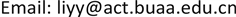1. 引言

· 我们提出一个非参数化的概念图模型，Biterm Topic Model with Dirichlet Process (BTMDP)，提取子事件的语义信息。

· 我们提出基于子事件语义信息的故事情节生成算法(LineGen)。

· 通过在三个数据集上的实验证明我们方法较已有方法的有效性。

2. 相关工作

3. 论文方法

3.1. 融合狄利克雷过程的词对主题模型(BTMDP)

3.1.1. BTMDP的概率图模型和生成过程

Cheng等人  提出一个对整个语料库上词共现模式的生成过程建模的词对主题模型(BTM)。BTM需要提前指定主题数。BTM的时间复杂度为O (K|B|)。主题数K越大，模型需要的时间越多。主题数越小，主题的粒度越粗。最好的方式是模型自动学习合适的主题数。基于这个想法，我们提出一个融合狄利克雷过程的词对主题模型(BTMDP)。因为狄利克雷过程的特性，主题数不需要人工干预即可自动学习。BTMDP可以看成是BTM的无限扩展模型。BTM和BTMDP的概率图模型如图1所示。

Notations of symbol

B语料库中所有词对
B ¬ b语料库除去词对b的所有词对
B z , ¬ b主题z的除去词对b的所有词对
Z各词对的主题值
Z ¬ b除去词对b的各词对的主题值
M词库中词的数量
n z主题z的词对数
n w | z主题z下词w的出现次数
n tBTMDP学到的主题数

z b | θ ∼ M u l t i ( θ ) (2)

ϕ k | β ∼ D i r ( β ) (3)

b | z b , { ϕ } k = 1 ∞ ∼ p ( b | ϕ z b ) (4)

3.1.2. BTMDP的推断过程

b是由两个词( w i , w j )组成的词对。 p ( b | ϕ z b ) = p ( w i | ϕ z b ) p ( w j | ϕ z b ) 。与BTM相同，BTMDP没有直接对文章的生成过程建模。在主题学习过程我们不能直接推断文章的主题分布。Yan等人  认为文章的主题分布等于文章生成的词对的主题分布的期望。即：

P ( z | d ) = ∑ b P ( z | b ) P ( b | d ) (5)

P ( z | b ) = P ( z ) P ( w i | z ) P ( w j | z ) ∑ z P ( z ) P ( w i | z ) P ( w j | z ) (6)

p ( z b = z | Z ¬ b , B , α , β ) ∝ p ( z b = z | Z ¬ b , B ¬ b , α , β ) p ( b | z b = z , Z ¬ b , B ¬ b , α , β ) ∝ p ( z b = z | Z ¬ b , α ) p ( b | z b = z , B z , ¬ b , β ) ∝ n z + α / K | B | − 1 + α ( n w i | z + β ) ( n w j | z + β ) ( ∑ w n w | z + M β ) ( ∑ w n w | z + M β + 1 ) (7)

p ( z b = z | Z ¬ b , B , α , β ) ∝ n z | B | − 1 + α ( n w i | z + β ) ( n w j | z + β ) ( ∑ w n w | z + M β ) ( ∑ w n w | z + M β + 1 ) (8)

p ( z b = K + 1 | Z ¬ b , B , α , β ) ∝ p ( z b = K + 1 | Z ¬ b , α ) p ( b | z b = K + 1 , β ) ∝ ( 1 − ∑ k = 1 K p ( z b = k | Z ¬ b , α ) ) p ( b | z b = K + 1 , β ) ∝ α | B | − 1 + α β 2 ( M β ) ( M β + 1 ) (9)

p ( w | z ) = n w | z + β ∑ w n w | z + M β (10)

p ( θ z ) = n z + α / n t | B | + α (11)

BTMDP的吉布斯抽样过程如算法1所示。因为空间限制，我们略去了吉布斯抽样的详细推导。原理和推导过程请参考   。

3.2. 故事情节生成(LineGen)

c e n t r o i d = ( c e n 1 , c e n 2 , ⋯ , c e n K ) c e n i = ∑ s ∈ s e l i s t s i ∑ m = 1 K c e n m (12)

s i = { s i ∑ j ∈ t s e s j i ∈ t s e 0                     others (13)

4. 实验和评价

4.1. 数据集和注解

4.2. 实验结果与分析

The example that some subevents are closer than others in a storylin
Se_idDescription
se1希拉里选择弗吉尼亚州联邦参议员蒂姆·凯恩（Tim Kaine）为副总统竞选搭档
se2特朗普宣布彭斯为竞选搭档
se3从希拉里和特朗普的民意调查来看，很难预测谁是赢
se4美大选希拉里支持率全面领先，特朗普被批有偏见

Annotation statistic

DS22基于关键词萨德和亲信干政门标注的85个子事件

4.2.1. 评价指标

P r e c i s i o n K = ∑ l = 1 L | C l ∩ t o p K ( C l ) | ∑ l = 1 L | t o p K ( C l ) | R e c a l l K = ∑ l = 1 L | C l ∩ t o p K ( C l ) | ∑ l = 1 L | C l | (14)

4.2.2. 对比方法

· DBSCAN：该方法用DBSCAN对子事件聚类。一个簇称为一个故事情节。

· NMF + DBSCAN：该方法首先用NMF生成子事件的嵌入表示，然后基于嵌入表示用DBSCAN对子事件聚类。一个簇称为一个故事情节。

· BTM + DBSCAN：该方法首先用BTM生成子事件的主题嵌入表示，然后基于主题嵌入表示用DBSCAN对子事件聚类。一个簇称为一个故事情节。

· BTMDP + DBSCAN：该方法首先用BTMDP生成子事件的主题嵌入表示，然后基于主题嵌入表示用DBSCAN对子事件聚类。一个簇称为一个故事情节。

· NMF + LineGen：该方法首先用NMF生成子事件的主题嵌入表示，然后基于主题嵌入表示用LineGen生成故事情节。

· LDA + LineGen：该方法首先用LDA生成子事件的主题嵌入表示，然后基于主题嵌入表示用LineGen生成故事情节。

· BTM + LineGen：该方法首先用BTM生成子事件的主题嵌入表示，然后基于主题嵌入表示用LineGen生成故事情节。

LDA + DBSCAN将所有的子事件聚到一个簇，我们展示LDA + DBSCAN的效果。在LDA、BTM和BTMDP中，吉布斯抽样迭代1000次。在需要指定主题数的NMF、LDA和BTM中，我们设定主题数为250。在LDA、BTM和BTMDP中，参数 α 和 β 分别为500和0.02。在NMF + DBSCAN、BTM + DBSCAN和BTMDP + DBSCAN中，我们使用的距离函数如式(15)所示。在NMF + LineGen、BTM + LineGen和BTMDP + LineGen中，我们使用的距离函数如式(16)所示。在LineGen中我们设置参数top-k为5。所有的结果是十次结果的平均值。

d i s ( s e 1 , s e 2 ) = 1 − cos ( s e 1 , s e 2 ) (15)

d i s ( p 1 , p 2 ) = 1 − 0.7 * J a c c a r d ( p 1 , p 2 ) − 0.3 * cos ( p 1 , p 2 ) (16)

4.2.3. 结果与分析

5. 工作总结与展望